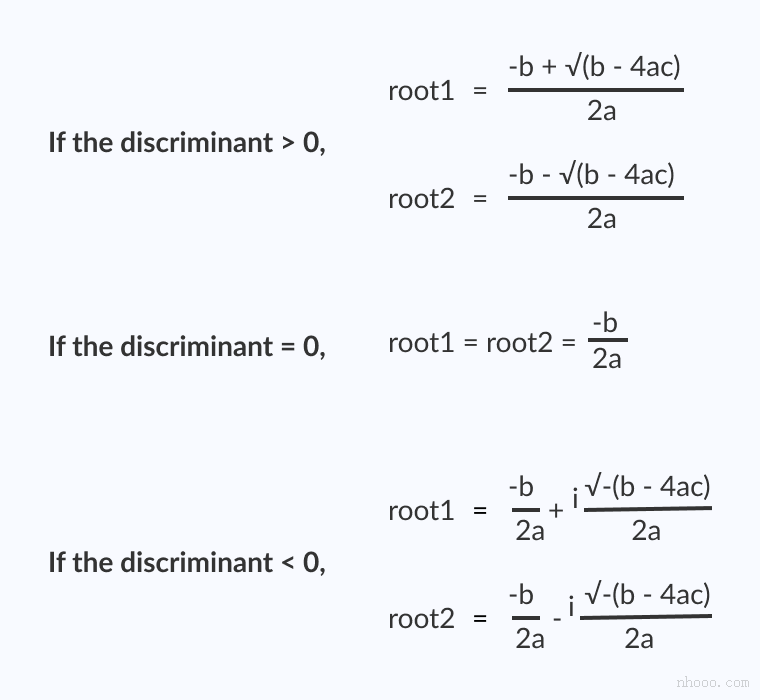• 2020-12-03 18:18:45

/*无聊时候写的，我想每个入门的编程都会拿着个练手把，多的不说，我们来谈谈正经的——此功能实现一元二次方程求解，复数情况，输入字符处理判断是否为数字*/

import os

import math

import cmath

/*自定义mx函数，求解，*/

def mx(a,b,c):

mm = (b ** 2) - (4 * a * c)

if mm > 0:

print('此函数有两个解')

x1=(-b+math.sqrt(mm))/(2*a)

x2 = (-b - math.sqrt(mm)) / (2 * a)

print("{:.0f}x**2+{:.0f}x+{:.0f}的结果为：x={:.0f} 和 x={:.0f}".format(a,b,c,x1,x2))

elif mm ==0:

print('此方程只有一个解')

print("{:.0f}x**2+{:.0f}x+{:.0f}的结果为：x={:.0f}".format(a, b, c, (-b+math.sqrt(mm))/(2*a)))

elif mm <0:

x1=(-b+cmath.sqrt(mm))/(2*a)

x2 = (-b - cmath.sqrt(mm)) / (2 * a)

print("{:.0f}x**2+{:.0f}x+{:.0f}的结果为：x={:.0f} 和 x={:.0f}".format(a, b, c, x1, x2))

else :

print('无解')

/*自定义is_number函数，判断用户输入的字符类型是否为数字，是为true不是为false。*/

def is_number(s):

try:

float(s)

return True

except ValueError:

pass

try:

import unicodedata

unicodedata.numeric(s)

return True

except (TypeError, ValueError):

pass

return False

/*自定义panduan函数，如果用户输入的字符类型为数字，返回数字，如果不是数字，则提示要求重新输入知道输入的为字符为止，(提示这里用while来实现循环，如果用户输入的一直不是数字，将会一直走不出while,最好用or一下用户输入的最大次数，即是用户输入的最大次数)。*/

def panduan(xx):

if is_number(xx)==True:

return xx

else:

while is_number(xx)==False:

print('不是数字，请再次输入')

cc =input("请输入一个数:")

if is_number(cc)==True:

break

return cc

/*主程序——input输入数字，先用is_number判断是否为数字，如果不是，返回false，再用panduan来再次依靠is_number的值，来进行判断为false则要求用户重新输入，为true则返回用户输入的数字，其中在python中input的数字为字符型，需要用float来转换成数字。 */

print('此程序用于计算一元二次方程解,请依次输入三个数')

zz=input("是否要开始计算:yes/任意退出:")

while zz =='yes':

a=float(panduan(input("请输入第一个数:")))

b=float(panduan(input("请输入第二个数:")))

c=float(panduan(input("请输入第三个数:")))

mx(a,b,c)

zz = input("是否还要继续计算:yes/no——:")

if zz!='yes':

qq =input('确认退出计算界面？yes/任意键继续')

if qq == 'yes':

print('感谢使用。' ,end="")

else:

zz=input("是否要开始计算:yes/任意退出:")

else:

zz = input("是否继续计算:yes/no——:")

更多相关内容
• 羽墨志记录一切开心与不开心目录⊙一元二次方程⊙判别式⊙求根公式⊙代码实现⊙运行结果前两天在《一元二次方程实数根的求解》一文中给出了一元二次方程在实数范围内的求解方法，换言之，方程在复数范围内的求解方法...羽墨志

记录一切开心与不开心目录

⊙一元二次方程

⊙判别式

⊙求根公式

⊙代码实现

⊙运行结果

前两天在《一元二次方程实数根的求解》一文中给出了一元二次方程在实数范围内的求解方法，换言之，方程在复数范围内的求解方法还未给出，今天就来填补一下前两天留下的坑吧。毕竟出来混迟早是要还的！

1

一元二次方程

百度百科

只含有一个未知数(一元)并且未知数项的最高次数都是2(两次)的整式方程叫作一元二次方程。一元二次方程经过整理都可化成一般形式ax²+bx+c=0(a≠0)。其中，ax²叫作二次项，a是二次项系数；bx叫作一次项，b是一次项系数；c叫作常数项。

——再复习一下概念

2

判别式

判别式还是那个熟悉的判别式：

①当△>0时，方程有两个不相等的实数根；

②当△=0时，方程有两个相等的实数根；

③当△<0时，方程无实数根，但有2个共轭复根。

(其中，△=b²-4ac，a、b、c分别是一元二次方程的二次项系数、一次项系数以及常数项。)

3

求根公式

△=b²-4ac<0时，一元二次方程稍微有了点变化：

在△=b²-4ac<0时，将a、b、c的值带入求根公式：就能计算得到一元二次方程在复数范围内的解。

4

代码实现/*** @file         :  equation.c* @author : Kevin* @version : 1.1.0.* @since        :  2019年7月23日18:20:17* @description : 求解一元二次方程*/#include #include #include /*** @function : main* @author : Kevin* @version : 1.1.0* @since : 2019年7月19日21:35:50* @description : main函数* @parameter : None* @return       :  None*/void main(int argc, char * argv[]){double a=0.0, b=0.0, c=0.0; //方程系数double delta = 0.0; //判别式double x1=0.0, x2=0.0; //方程的实根double real=0.0; //实部double imag=0.0; //虚部printf("Input the coefficient of equation:a,b,c\n");scanf("%lf,%lf,%lf",&a,&b,&c);fflush(stdin); //清空输入流缓冲区中的内容if(a != 0){delta = pow(b,2)-4*a*c;//△>0，方程有两个不等实根if(delta>0){x1 = (-b+sqrt(delta))/(2.0*a);x2 = (-b-sqrt(delta))/(2.0*a);printf("△=%.2f>0, the equation has two different real roots:\n",delta);printf("x1=%.2f, x2=%.2f.\n",x1,x2);}else if(delta==0)//△=0，方程有两个相等实根{x1=x2=-b/(2*a);printf("△=%.2f=0, the equation has two equal real roots:\n",delta);printf("x1=x2 = %.2f.\n",x1);}else//△>0，方程在实数范围内无解，但有两个共轭复数根{printf("△=%.2f<0, the equation has two complex conjugate roots:\n",delta);real = -b/(2.0*a);imag = sqrt(-delta)/(2.0*a);//输出两个共轭复数根if(imag>0){printf("x1=%.2f+%.2fi, x2=%.2f-%.2fi.\n",real,imag,real,imag);}else{printf("x1=%.2f%.2fi, x2=%.2f+%.2fi.\n",real,imag,real,-imag);}}}else //a=0,一元一次方程并给出解{printf("The equation is not a binary primary equation!\n");printf("Solution of the equation is %.2f.\n",-b/c);}}

5

运行结果时间改变了我们的样子

却在心里留下了彼此的影子26

星期五

2019年7月

想和你重新认识一次，从你叫什么名字说起。

——新海诚《你的名字》

一日一签

展开全文• C程序求二次方程在此示例中，您将学习如何在C语言编程中找到二次方程。要理解此示例，您应该了解以下C语言编程主题：二次方程的标准形式为：ax2+bx+c=0,当a,b和c是实数，a!=0b2-4ac术语被称为二次方程的判别...

C程序求二次方程的根

在此示例中，您将学习如何在C语言编程中找到二次方程的根。

要理解此示例，您应该了解以下C语言编程主题：

二次方程的标准形式为：ax2 + bx + c = 0, 当

a, b 和 c 是实数，

a != 0

b2-4ac术语被称为二次方程的判别式。它说明了根的性质。如果判别式大于0，根是不同的实数

如果判别式等于0，则根是实数且相等。

如果判别式小于0，则根是不同的复数。查找二次方程根的程序#include

#include

int main() {

double a, b, c, discriminant, root1, root2, realPart, imagPart;

printf("输入系数a，b和c: ");

scanf("%lf %lf %lf", &a, &b, &c);

discriminant = b * b - 4 * a * c;

// 实数根不等的条件

if (discriminant > 0) {

root1 = (-b + sqrt(discriminant)) / (2 * a);

root2 = (-b - sqrt(discriminant)) / (2 * a);

printf("root1 = %.2lf and root2 = %.2lf", root1, root2);

}

// 实数根相等的条件

else if (discriminant == 0) {

root1 = root2 = -b / (2 * a);

printf("root1 = root2 = %.2lf;", root1);

}

// 如果根不是实数

else {

realPart = -b / (2 * a);

imagPart = sqrt(-discriminant) / (2 * a);

printf("root1 = %.2lf+%.2lfi and root2 = %.2f-%.2fi", realPart, imagPart, realPart, imagPart);

}

return 0;

}

输出结果输入系数a，b和c: 2.3

4

5.6

root1 = -0.87+1.30i and root2 = -0.87-1.30i

在此程序中，sqrt()库函数用于查找数字的平方根。要了解更多信息，请访问：sqrt()函数。

展开全文• 一元二次方程 ax²+bx+c=0 的包含3种情况(这里仅讨论a!=0的情况) #include<stdio.h> #include<math.h>void ax(int a,int b,int c); //δ>0void bx(int a,int b,int c); //δ=0void cx(int a,int b,...

一元二次方程 ax²+bx+c=0 的根包含3种情况
Δ>0
Δ=0
Δ<0
(这里仅讨论a!=0的情况)

#include<stdio.h>
#include<math.h>
void ax(int a,int b,int c); //Δ>0
void bx(int a,int b,int c); //Δ=0
void cx(int a,int b,int c); //Δ<0
int main()
{
int a,b,c;
scanf("%d%d%d",&a,&b,&c);
if(a<0) {
a=-a;
b=-b;
c=-c;
}
if(b*b-4*a*c>0)
ax(a,b,c);
if(b*b-4*a*c==0)
bx(a,b,c);
if(b*b-4*a*c<0)
cx(a,b,c);
return 0;
}
void ax(int a,int b,int c) {
double x0,x1,x2;
x0 = sqrt(b*b-4*a*c);
x1=(-b+x0)/(2.0*a);
x2=(-b-x0)/(2.0*a);
printf("x1=%.3f x2=%.3f\n",x1,x2);
}

void bx(int a,int b,int c) {
double x = -b/(2.0*a);
printf("x1=%.3f x2=%.3f\n",x,x);
}

void cx(int a,int b,int c) {

double m = -b/(2.0*a);
double n=sqrt(-(b*b-4*a*c))/(2.0*a);
printf("x1=%.3lf+%.3lfi x2=%.3lf-%.3lfi\n",m,n,m,n);
}


展开全文• 可以计算一元二次方程复数根的模拟计算机 模拟计算机采用模拟电路计算加减乘除。例如计算，（28+80-65）×56÷79，可以先用直流电源电路产生一个DC28V,1A的信号，这个信号代表实数28。再用直流电源电路产生一个DC80V...
• "" Text2.Text = "" '清空用户名和密码等待重新输入 Text3.Text = "" Text4.Text = "" Text5.Text = "" Label15.Caption = ""End IfIf m >= 0 Then X1 = (-b + Sqr(m)) / (2 * a) '自定义一元二次方程求根函数 ...
• a=0,不是一元二次方程； b^2-4ac=0,有两相等的实根；x1=x2=-2a/b;b^2-4ac>0,有两不等的实根；p=-b/2a,q=sqrt(b^2-4ac)/2a;x1=p+q,x2=p-q;b^2-4ac<0,有两个共轭复根；p=-b/2a,q=sqrt(4ac-b^2)/2a;x1=p+qi,x2=p-...
• 提高题 1) 设有一元二次方程如下: aX bx c=0 试根据从键盘输入的 a,b,c 求解方程的。 【提示】要考虑各种情形:一是 a 为0的情形,方程为-c/......(理论考试重点内容实践) 一元二次方程白盒测试要求 1) 自备 C ...
• 共回答了15个问题采纳率：80%import java.util.*;class First{public static void main(String[] args)...System.out.println("请输入一元二次方程的三个系数a,b,c的值");System.out.println("请输入系数a的值");dou...
• 初中二次函数求根公式是什么2020-10-03 14:51:04文/宋则贤一般地，把形如y=ax²+bx+c(a≠0)(a、b、c是常数)的函数叫做二次函数，下面总结了二次函数的相关知识点，供大家参考。初中二次函数求根公式二次函数的求根...
• 求解一元二次方程的思路 求解一元二次方程 ax2+bx+c=0ax^2+bx+c=0ax2+bx+c=0 的思路，将该方程转化为 (x−x′)2=K(x-x')^2=K(x−x′)2=K，得到 x=±K+x′x = \pm\sqrt{K}+x'x=±K​+x′ 所以有如下转化过程： ax2+bx...数学
• 题目： 从键盘输入三个实数，输出实数复数根 代码： #include <stdio.h> #include <...void root(double a,double b,double c);... printf("请输入一元二次方程x-y-z的值："); scanf("%lf,%...
• 一元二次方程ax2+bx+c=0，输入a,b,c三个系数，求解方程，结果有三种情况：两个实数、一个实数、两个复数根。/*** Equation.java*/package cn.edu.uibe.oop;/*** 计算一元二次方程* @author TongQiang**/...
• 利用C++一元二次方程，不能单纯地依靠数学原理，否则会得出错误的结果！ 先看一道例题： 1058：一元二次方程 时间限制: 1000 ms 内存限制: 65536 KB 提交数: 102128 通过数: 18399 【题目描述】 利用...c++ 开发语言 后端 c语言
• import math a = float(input("请输入a的值：")) b = float(input("请输入b的值：")) c = float(input("请输入c的值：")) if a != 0: delta = b**2-4*a*c if delta <... print("唯一x=",s) else : ...python python
• } 输出示例 分析 注意无实根情况的判定，且注意和恰好相切情况的处理。比如68行的sqrt(epsilon)可以想想为什么要加根号。 一开始第3张输出图片输出-0，经过调整，使-0变为0。关于-0和0详见我的博客 关于 C++中 -0和...c++
• 本文使用Python实现一元二次方程求根公式，主要演示运算符和几个内置函数的用法，封面图片与本文内容无关。def root(a, b, c, highmiddle=True): #首...python 人工智能 机器学习
• 编写程序求解一元二次方程（ax^2 + bx + c = 0)的： 描述算法： 1.创建a, b, c三个变量，从键盘获取这三个变量的值； 2.计算delta； 3.根据delta判断方程的 当delta>=0 ： 方程有两个不相等的 x1 = (-b + ...
• 一元二次方程ax2+bx+c=0，输入a,b,c三个系数，求解方程，结果有三种情况：两个实数、一个实数、两个复数根。/*** Equation.java*/package cn.edu.uibe.oop;/*** 计算一元二次方程* @author TongQiang**/...
• 该楼层疑似违规已被系统折叠隐藏此楼查看此楼#include #include #include using namespace std;void fun(double a,double b,double c){double det;... "这不是二次方程" << endl;return ;}det = ...
• 本题目要求读入3个一元二次方程的系数a,b,c,出该方程的（也可能有复数根）。 解题提示：Python的 math模块 中 sqrt 函数负数平方根会报错， 因为math 模块中 sqrt 函数只能进行浮点数的运算。我们知道负数的...
• print("根据a*x^2+b*x+c=0,在a≠0的情况下，有两个，x1=（-b+√（b^2-4ac）/2a和x2=(-b-√(b^2-4ac))/2a）\n2x^2+3x+1=0的是多少") while 1: try: a,b,c=input("输入a、b、c对应的三个数，用空格隔开,a≠0：")...
• 计算机语言运用--数值计算9-方程的计算机处理92-4_Fortran数值计算方程的计算机处理这里说的方程，是以实数域为计算范围。这里说的方程：是含有未知数的等式。可以是a+5=8，或X+A=B，或ax2+bx+c=0，或ax+by=c与dx-ey...
• 满意答案JJ宋娇2017.01.09采纳率：54%等级：8已帮助：813人#include "stdio.h"#include "math.h"int main(void){int a,b,c;double d,e,f;scanf("%d %d %d",&...if (a==0)if (b==0){printf("非法方程，请...
•python 开发语言 后端
• 前言 提示：这里可以添加本文要记录的大概内容： 例如：随着人工智能的不断发展，机器学习这门技术也越来越重要，很多人都开启了学习机器学习，本文就介绍了机器学习的基础内容。... //定义方程系数及 scan...